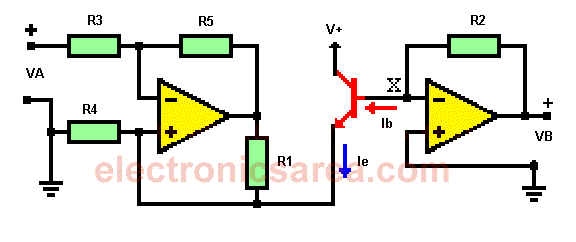Circuit for measuring beta of transistor

The beta of transistor is the amplification factor between the base current and the collector current. We can obtain  beta of  transistor (β) using a voltage-current converter and current-voltage converter.

Beta (β) is a characteristic of each transistor. This feature is found in the NTE, ECG manuals, etc.. These manuals have a minimum or approximate values of the real values. This means that we do not know the real beta of transistor.

Keep in mind that two transistors with the same name (eg 2N2222) may have different betas. The circuit above allows us to obtain the value of beta, for a specific transistor.How the circuit for measuring beta of transistor works?

This circuit comprises a voltage to current converter, on the left side of the transistor and a current to voltage converter, on the right side (see diagram). The voltage to current converter controls the emitter current of the transistor and the current-voltage converter controls the base current of the transistor.

The current-voltage converter is easily implemented, with an inverting amplifier that does not use an input resistor. The base current flows through the virtual ground (point X), whose voltage is not affected by the current, while the output VB is proportional to this current (Ib), at the input of operational amplifier.

The part of the circuit which controls the emitter current the current voltage converter and supplies the emitter current of the transistor. The base of the transistor is 0 V. (virtual earth between the inverter and noninverting terminals of operational amplifier) so that the voltage on the emitter is -Vbe.

The emitter current is set with the input voltage of the voltage-current converter and the resulting current (base) is obtained by measuring the output voltage of the current-voltage converter.

Beta (β) = 1 + Ie/Ib. As Ie = VA/R1 and Ib = VBR2

Beta (β) = 1 + VA/R1 x R2/VB = 1 + [VAxR2] / [VBxR1]

With R1 = R4 = 1k, R2 = R3 = R5 = 100K, Beta (β) = 1 + [VA x 100K] / [VB x 1K].

As Ven=VA, the beta of transistor (β) is obtained using the formula: Beta (β) = 1+100 Vent/VB.

•
•
•
•
•
•
•
•
•
•
•
•
•
•
•
•
•
•
Tagged with: